## Policy Gradient

### 基本思想

Policy Gradient 就是通过更新 Policy Network 来直接更新策略的。那什么是 Policy Network？实际上就是一个神经网络，输入是状态，输出直接就是动作（不是Q值），且一般输出有两种方式：一种是概率的方式，即输出某一个动作的概率；另一种是确定性的方式，即输出具体的某一个动作。

$L(\theta) = \mathbb E(r_1+\gamma r_2 + \gamma^2 r_3 + …|\pi(,\theta))$

$L(\theta) = \sum log\pi(a|s,\theta)f(s,a)$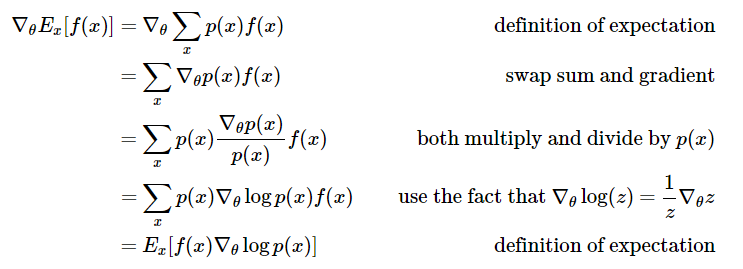### 评判指标的选择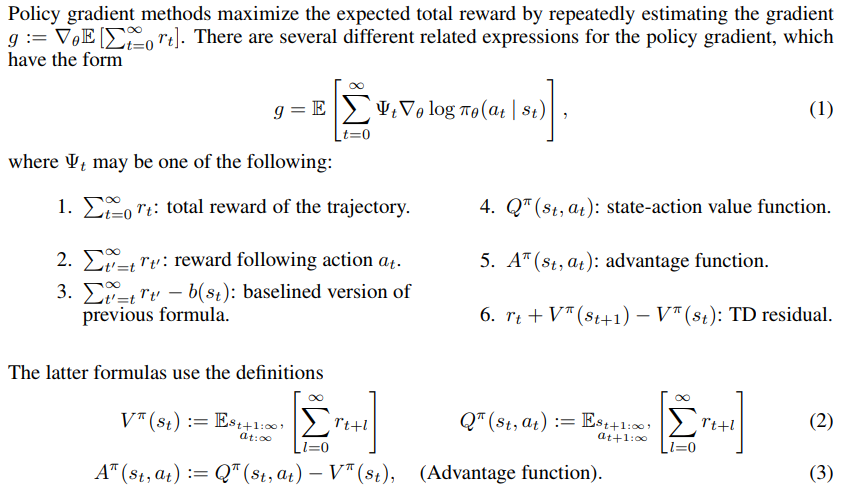## Actor-Critic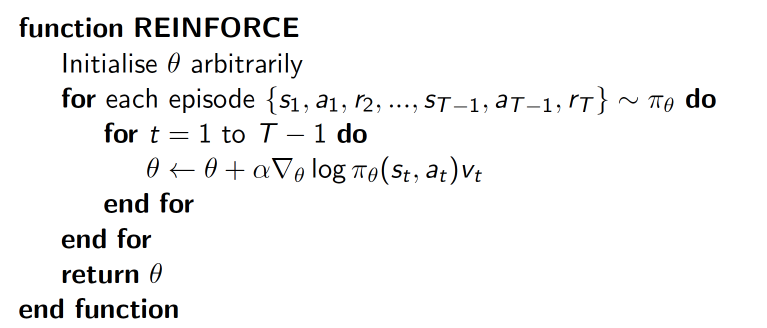## Deep Deterministic Policy Gradient(DDPG)

DDPG 相对于 DPG 的核心改进是引入了 Deep Learning，采用深度神经网络作为 DPG 中的策略函数 $μ$ 和 $Q$ 函数的模拟，即 Actor 网络和 Critic 网络；然后使用深度学习的方法来训练上述神经网络。两者的关系类似于 DQN 和 Q-learning 的关系。

DDPG 的网络结构为 Actor 网络 + Critic 网络，对于状态 $s$, 先通过 Actor 网络获取 action $a$, 这里的 $a$ 是一个向量；然后将 $a$ 输入 Critic 网络，输出的是 Q 值，目标函数就是极大化 Q 值，但是更新的方法两者又有一些区别。论文中显示 DDPG 算法流程如下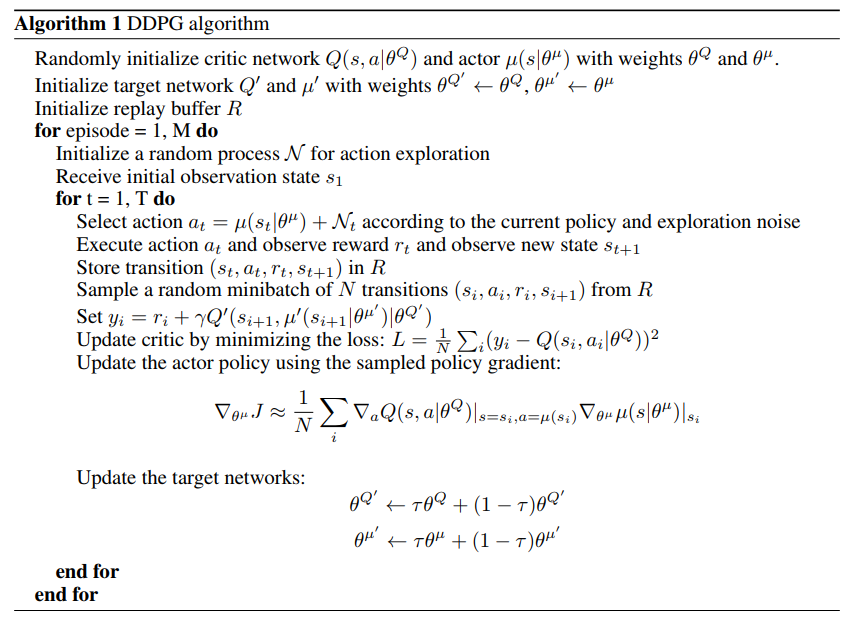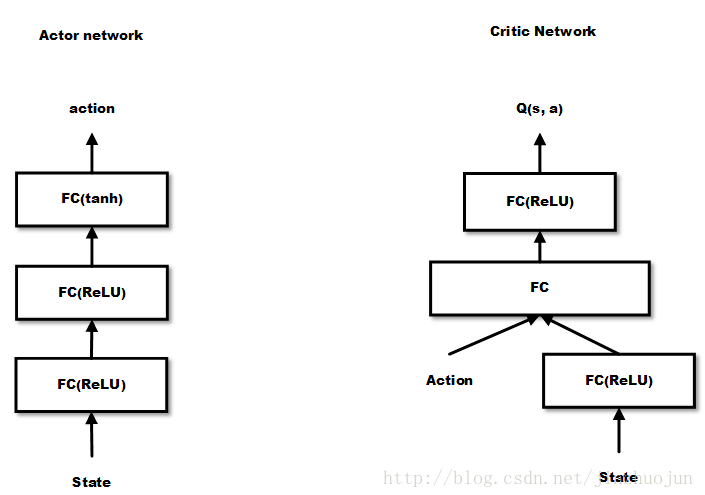## Asynchronous Advantage Actor-Critic(A3C)

A3C 算法和DDPG类似，通过 DNN 拟合 policy function 和 value function的估计。但是不同点在于

1. A3C 中有多个 agent 对网络进行 asynchronous update，这样带来了样本间的相关性较低的好处，因此 A3C 中也没有采用 Experience Replay 的机制；这样 A3C 便支持 online 的训练模式了
2. A3C 有两个输出，其中一个 softmax output 作为 policy $\pi(a_t|s_t;\theta)$，而另一个linear output为 value function $V(s_t;\theta_v)$
3. A3C 中的Policy network 的评估指标采用的是上面比较了多种评估指标的论文中提到的 Advantage Function(即A值) 而不是 DDPG 中单纯的 Q 值。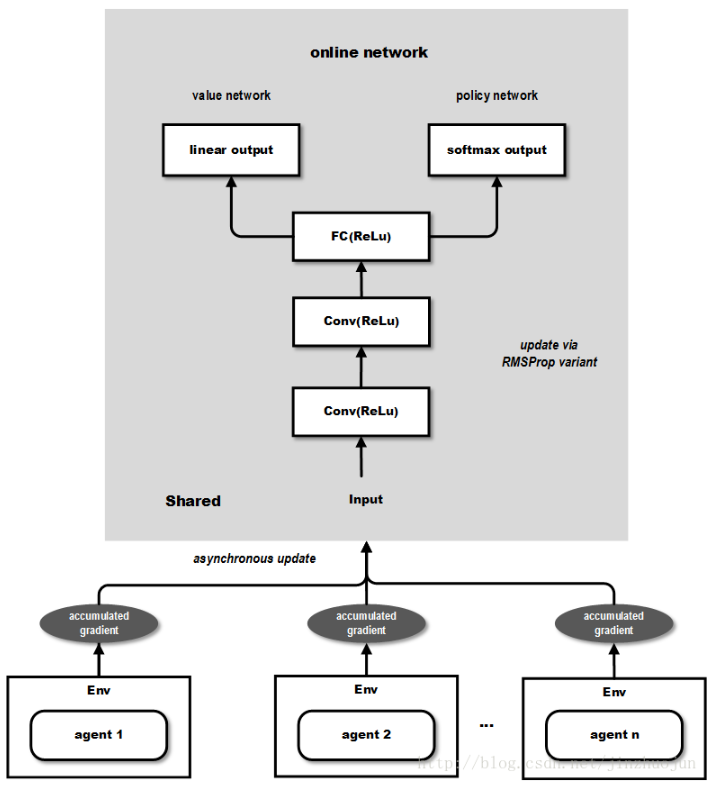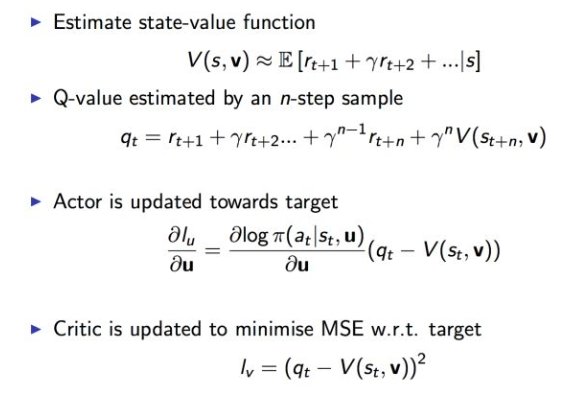A3C 通过创建多个agent，在多个环境实例中并行且异步的执行和学习，有个潜在的好处是不那么依赖于GPU或大型分布式系统，实际上A3C可以跑在一个多核CPU上，而工程上的设计和优化也是这篇文章的一个重点。